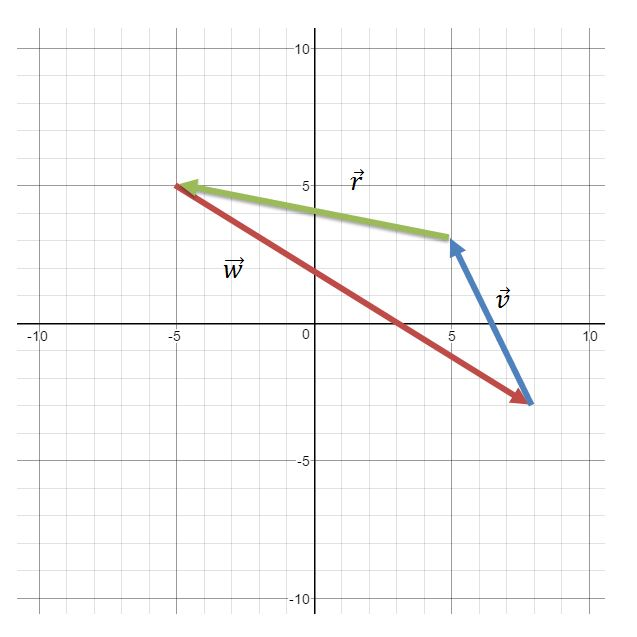# Introduction to vectors - Vectors

### Introduction to vectors

The notion of vectors can be easily found in our daily surroundings – from airplane navigation to trajectories of balls during a sports game, to movements of a character you maneuver in your video games. In this section, we will learn about the graphical, and mathematical representation of a vector, as well as how to distinguish the differences between a scalar quantity and a vector quantity.

#### Lessons

##### For the following vectors, express them in component form, matrix form, and rectangular form